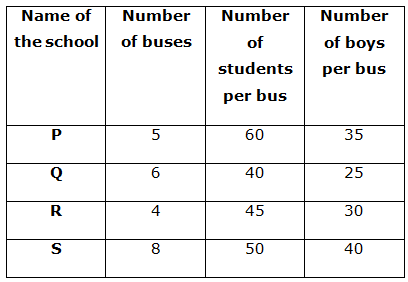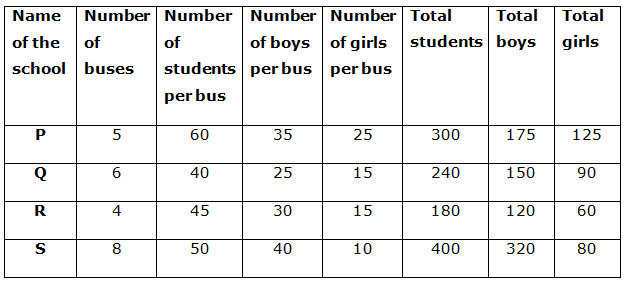# SBI PO Prelims Quantitative Aptitude Questions 2019 – Day 11

SBI PO 2019 Notification will be expected soon. It is one of the most expected recruitment among the banking aspirants. Every year the exam pattern for SBI PO has been changing. Depends upon the changing of exam pattern the questions are quite harder compare to the previous year. So the questions are in high level than the candidate’s assumption.

As per the latest trend, our IBPS Guide is providing the updated New Exam Pattern Quantitative Aptitude questions for SBI PO 2019 Day 11. Our Skilled experts were mounting the questions based on the aspirant’s needs. So candidates shall start your preparation and practice on daily basis with our SBI PO pattern quantitative aptitude questions 2019 day 11. Start your effective preparation from the right beginning to get success in upcoming SBI PO 2019.

[WpProQuiz 5141]

### Click Here for SBI PO Pre 2019 High-Quality Mocks Exactly on SBI Standard

Directions (1 – 5): Study the following graph carefully and answer the given question.

The table shows the number of students per bus in four different schools.1) Total number of boys in all the buses in school R is what percentage more/less than the total number of boys in all the buses in school Q?

a) 10%

b) 24%

c) 20%

d) 25%

e) 30%

2) Find the ratio of the total number of girls in all the buses in schools Q and R together to that of the total number of boys in all the buses in the same schools?

a) 4: 7

b) 7: 6

c) 5: 9

d) 3: 5

e) 2: 3

3) What is the difference between the total number of boys in all the buses in all the given schools together to that of total number of girls in all the buses in all the given schools together?

a) 328

b) 410

c) 290

d) 430

e) 370

4) In which of the following schools, the difference between the number of boys in all the buses and the number of girls in all the buses is maximum?

a) S

b) Q

c) P

d) R

e) None of these

5) Total number of students in all the buses in schools P and R together is what percentage of the total number of students in all the buses in schools Q and S together?

a) 80%

b) 60%

c) 45%

d) 75%

e) 50%

Directions (6 – 10): What approximate value should come at the place of question mark in the following questions?

6) √2400.998 x 19.001 – √676.012 x 5.99 + 45.9 x 6.86 = ?

a) 989

b) 1125

c) 1307

d) 1289

e) 1097

7) 4590.099 ÷ 5.012 + 359.91 x 66.87 – 1089.99 =?

a) 24424

b) 23948

c) 25122

d) 20142

e) 18642

8) 8.9% of 899.999 + 55% of 6000.0156 – 14.79% of 1199.898 =?

a) 987

b) 3201

c) 2119

d) 1605

e) 4424

9) 3√9260.99÷ 6.99 + √5776.11 ÷ 4.02 – 5.99 x 3.4 =?

a) 10

b) 15

c) 4

d) 9

e) 6

10) 7989.669 – 322.004 + 912.0089 – 9.999 + 89.99 =?

a) 8660

b) 7540

c) 6120

d) 5440

e) 4980

Directions (1 – 5):Total number of boys in all the buses in school R = 4 * 30 = 120

Total number of boys in all the buses in school Q = 6 * 25 = 150

Required percentage = [(150 – 120)/150] * 100

= 30/150 * 100

= 1/5 * 100

= 20%

Required ratio = (90 + 60): (150 + 120)

= 150: 270

= 5: 9

Total number of boys in all the buses in all the given schools

= 175+150+120+320

= 765

Total number of girls in all the buses in all the given schools

= 125 + 90+ 60 + 80

= 355

Required difference = 765 – 355 = 410

Difference between the number of boys and girls in all the buses in school P

= 175 – 125 = 50

Difference between the number of boys and girls in all the buses in school Q

= 150 – 90 = 60

Difference between the number of boys and girls in all the buses in school R

= 120 – 60 = 60

Difference between the number of boys and girls in all the buses in school S

= 320 – 80 = 240

School S has maximum difference between the number of boys and number of girls.

Required percentage = [(300+180) / (400+240)] * 100

= 480/640 * 100

= 75%

Direction (6-10) :

√2400.998 x 19.001 – √676.012 x 5.99 + 45.9 x 6.86 = ?

=>√2401 x 19 – √676 x 6 + 46 x 7 =?

=> 49 x 19 – 26 x 6 + 322 =?

=> 931 – 156 + 322 =?

=> 1097 =?

4590.099 ÷ 5.012 + 359.91 x 66.87 – 1089.99 =?

=> 4590 ÷ 5 + 360 x 67 – 1090 =?

=> 918 + 24120 – 1090 =?

=> 23948 =?

8.9% of 899.999 + 55% of 6000.0156 – 14.79% of 1199.898 =?

=> 9% of 900 + 55% of 6000 – 15% of 1200 =?

=> (9/100) x 900 + (55/100) x 6000 – (15/100) x 1200 =?

=> 81 + 3300 – 180 =?

=> 3201 =?

3√(9260.99) ÷ 6.99 + √5776.11 ÷ 4.02 – 5.99 x 3.4 = ?

=> ∛9261÷ 7 + √5776 ÷ 4 – 6 x 3 =?

=> 21 ÷ 7 + 76 ÷ 4 – 18 =?

=> 3 + 19 – 18 =?

=> 4 =?